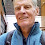### Have Climate Projections Been Accurate?

I went through some of the most well-known climate projections from decades ago and compared them with actual observations to test their accuracies.

#### Projections checked

I chose the following projections to test:
It takes a long time to scan these reports and get the data, so I stopped after these. The IPCC is the most well-accepted summary and the Hansen report is cited often, so these felt like good ones to use and I searched them first. I didn't use more recent ones simply because there hasn't been enough time...seeing how well a 2014 paper predicted temperatures in 2016 isn't as interesting as seeing how well a 1988 paper did.

#### Data to test against

For temperatures, I used the NASA data set. For sea levels, I used this page from climate.gov. For all observations, I used 5-year averages around the specified point. For example, a 1990 point is the average of 1988, 1999, 2000, 2001, and 2002.

#### Results

These plots can get really cluttered, so I wanted to make them as simple as possible. Thus, each plot just shows a high projection, low projection, and the observed value. If the observed value lies between the high and low, that's an indication that the projection was accurate.

To account for the difference in starting points for each projection in the initial year, I used the shift from one year to the other. For example...if the high estimate was 0.5 in 1990 and 1.5 in 2016, that's a 1 degree shift from 1990 to 2016. If the low estimate for the same data was 0 in 1990 and 0.5 in 2016, that's a 0.5 degree shift from 1990 to 2016.

I could just check the absolute accuracy of the estimates, but that is biased towards finding that the models were more accurate than they actually were. For example, with the numbers above, say that temperature was 0.4 in 1990 and 0.6 in 2016. The values in 1990 would be 0.0, 0.4, and 0.5. In 2016 they'd be 0.5, 0.6, and 1.5. This would seem like the projections were accurate. However, dealing with the shifts, the values would be 0.5, 0.2, and 1.0. The actual shift of 0.2 is outside the projected range of 0.5 to 1.0 and it would show that the projections were not accurate.

IPCC 1990 temperatures:

Here is the set of projections:

Here is how they compare with subsequent observations:

The 'best' projection there is a rise of 0.56 degrees while the observed change was 0.51 degrees.

1988 Hansen temperatures

Here is the set of projections:

Here is how they compare with subsequent observations:

Hansen's plot was straight projections rather than a giant spread. Scenarios A (exponential rise), B (maintain historic rise), and C (emissions reductions starting around 2000) were various emissions projections. Scenario B was closest to the actual emissions that happened. It predicted a rise of 0.6 degrees while the observed change was 0.51 degrees.

IPCC 2001 temperatures:

Here is the set of projections:

Here is how they compare with subsequent observations:

The high and low here are the wide shaded area. Taking the thick, black line as the 'best' projection, it projected a rise of 0.23 degrees while the actual rise was 0.34 degrees.

IPCC 1990 sea level

Here is the set of projections:

Here is how they compare with the subsequent observations:

The 'best' projection there is a rise of 0.10 m while the observed change was 0.07 m.

IPCC 2001 sea level

Here is the set of projections:

Here is how they compare with the subsequent observations:

The high and low rise here are the wide shaded area. Taking the thick, black line as 'best' here, it predicted a rise of 0.035 m vs an observed rise of 0.048 m.

#### Conclusion

All of the projections were accurate. The observations lied pretty close to the 'best' projection in each case except for the 2001 sea level rise projections in which the observed rise was very close to the high projection.

There is a lot of uncertainty here. I had to digitize these images manually, and that introduces some error. However, the error from that is much smaller than the separation between bars in all but the last image.

To be honest, I'm a bit surprised. Going into this, I'd guessed that the IPCC temperature projections were too low and that the observations would have been higher than the projections in general. I can't really argue with the results though so it looks like they were accurate.

1.Sea level rise is pretty scary. Overall, an impressive work. The media needs to do more work like this. Denialists often imply that climatologists are cooking the books.

1.Thanks. I tried searching for this sort of thing, and couldn't find these exact checks. I figured Scientific American or someone would have posted this with much more data and quality than I have here.

2.OUTSTANDING work.